Smartick is a fun way to learn math!Jun02

# How to Find a Sum of Fractions

In this post, we are going to learn how to find a sum of fractions.

Before you begin to add fractions, it is recommended that you know how to calculate the least common multiple (LCM) of two or more numbers.

To calculate a sum of fractions, the important thing is that the fractions have the same denominator.

## Sum of fractions with the same denominator

To add fractions with the same denominator you have to add the numerators and leave the same denominator.

For example: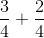Since the 2 fractions have the same denominator, what we have to do is keep the same denominator, which is 4, and add the numerators.

3 + 2 = 5

And the result of the sum of fractions is: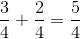## Sum of fractions with different denominators

To add fractions with different denominators, the first thing that you have to do is find a common denominator: this is the least common multiple of the denominators that you have. Then we multiply each numerator by the number that we have multiplied the denominator by. Finally, we add the numerators that we have obtained and keep the same denominator.

For example,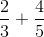The first thing to do is find a common denominator between 3 and 5. To do this, we calculate the least common multiple between both numbers.

LCM(3,5) = 15

So 15 is the common denominator of the two fractions.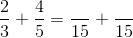Now we have to multiply each numerator by the number that we have multiplied the denominator by. To do this, we divide the LCM by the initial denominator and multiply the result by the numerator of that fraction.

For the first fraction:

15 / 3 = 5

5 x 2 = 10

So 10 is the numerator of the first fraction.

For the second fraction:

15 /  5 = 3

3 x 4 =12

So 12 is the numerator of the second fraction.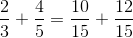Now, all we have left to do is add the numerators:

10 + 12 = 22

And the result of the sum of fractions is: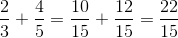I hope that you have learned with this post how to to find a sum of fractions.

Do not hesitate to leave your comments!

Fun is our brain’s favorite way of learning
Diane Ackerman
Smartick is a fun way to learn math
• 15 fun minutes a day
• Millions of students since 2009• joshuaMar 10 2021, 5:36 AM

Awesome

• mathisnotadancemoveDec 17 2020, 1:53 PM

Thanks Smartick…this really helped me a lot.

• BuvanNov 01 2020, 12:55 AM

Thank goodness

• chelsey gayle afallaOct 13 2020, 10:51 PM

Omg… I like it😍

• jjSep 29 2020, 1:30 AM

• scott corlessApr 03 2020, 4:42 AM

wow works soooo well thx smartick

• AdanechDec 20 2019, 8:25 AM

i enjoy with this

• jjSep 29 2020, 1:31 AM

I love it too, don´t give up!

• Horse girl 202202Mar 07 2019, 7:24 PM

Thanks, it really helped me with my homework

• julianna c. youngFeb 13 2019, 9:35 PM

Thank you for helping me with math. You make it so easy.

• Kat GJan 28 2019, 9:49 PM

OML This freaking helped me a lot! Thanks I will sure use your website again! 😀

• Samapti BagchiAug 25 2018, 11:48 AM

• sunnyFeb 14 2018, 7:57 AM

super easy to understand. love ittttttt. thank internet so much and smart people to make math easy.